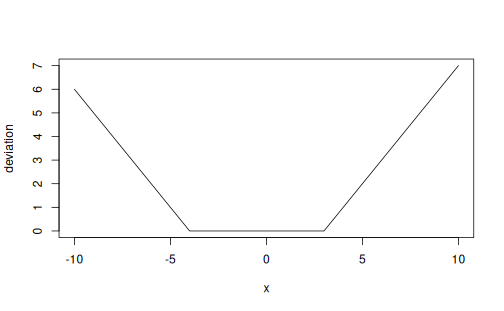dev {agvgd} R Documentation

## Deviation function

### Description

This function calculates the deviation in the sense of the Grantham deviation as introduced by Tavtigian et al. (2006). Essentially, if x lies within the range [min, max], then dev() returns 0. If x is either below min, or above max, then dev() returns the absolute difference between x and min or max, respectively.Inputs are recycled in the sense of vctrs::vec_recycle().

### Usage

dev(x, min, max)


### Arguments

 x A numeric vector. min A numeric vector. max A numeric vector.

### Details

Here's a plot showcasing dev() with min = -4 and max = 3:

x <- -10:10; min <- -4; max <- 3
plot(x, y = dev(x, min, max), type = 'l', xlab = 'x', ylab = 'deviation')### Value

A numeric vector of deviations.

gd()

### Examples

# dev() returns absolute differences from either min or max (whichever is
# closest).
dev(10, min = -4, max = 4)
dev(-10, min = -4, max = 4)

# x can be a vector
dev(-10:10, min = -4, max = 4)

# min and max can also be vectors, they will be recycled
dev(-10:10, min = -4:16, max = 4:24)

# If x contains NA values, then dev() will return NA for
# those cases
dev(c(10, NA), min = -4, max = 4)

# For each calculation of deviation, only either min or max is used. If
# the unused parameter is NA it won't affect the calculation:
dev(c(10, 3), min = c(NA, -4), max = 4)
dev(c(10, -5), min = -4, max = c(4, NA))



[Package agvgd version 0.1.2 Index]